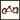# 小朋友才『寫』測試，成熟的大人都 Property-Based Testing

October 05, 2021

## 什麼是 `Property-Based Testing`？

`Property-Based Testing` 起源於 2000 年由 `Koen Claessen``John Hughes` 兩位教授所寫的 `QuickCheck: a lightweight tool for random testing of Haskell programs` 論文中所介紹的 `QuickCheck` 此 Haskell 的 testing framework。此後，也啟發各個語言的 `Property-Based Testing` framework，例如： Python 的 `Hypothesis`、Erlang 的 `PropEr`、Rust 的 `quickcheck`、JS/TS 的 `fast-check` 等等。

`Property-Based Testing` 有什麼優缺點呢？為什麼各語言都爭相做出該語言的 `Property-Based Testing` 框架呢？

※ 本文將使用 JS/TS 的 `Property-Based Testing` 框架 `fast-check` 做範例來介紹如何透過 `Property-Based Testing` 做測試。

### Don’t write tests!

John Hughes 在 Curry On! 2017 給了一場演講名為 `Don't Write Tests!`。為什麼他會下這種標題呢？讓我們來舉例並逐步了解。

``````describe(`Test \${reverse.name}`, () => {
it('should reverse the array', () => {
expect(reverse([1, 2, 3])).toEqual([3, 2, 1]);
expect(reverse([1, 2, 3, 4, 5])).toEqual([5, 4, 3, 2, 1]);
});
});

describe(`Test \${sort.name}`, () => {
it('should sort the array in ascending order', () => {
expect(sort([1, 3, 5, 4, 2])).toEqual([1, 2, 3, 4, 5]);
expect(sort([2, 1, 3, 4])).toEqual([1, 2, 3, 4]);
expect(sort([5, 4, 3, 2, 1])).toEqual([1, 2, 3, 4, 5]);
});
});``````

### Example-Based Testing 的問題是什麼？

``````function range(n: number): Array<number> {
return Array.from({ length: n }).map((_, i) => i + 1);
}

describe(`Test \${sort.name}`, () => {
it('should sort the array in ascending order', () => {
expect(sort(range(3).reverse())).toEqual(range(3));
expect(sort(range(5).reverse())).toEqual(range(5));
expect(sort(range(10).reverse())).toEqual(range(10));
});
});``````

`range` function 可以產生一個由 1 到 N 的陣列，但由於他已經是一個排序好的陣列，我們必須先透過一些方法（此範例使用 `.reverse`）來打亂陣列順序後，再透過執行 `sort` function 來確認是否已經排序好了。這樣的優點是，我們已經可以透過產生出的陣列來測試，並打亂它後再確認是否已經排序，但是，這樣我們還是只有測了「長度為 3 且內容物為 1 到 3 的陣列」、「長度為 5 且內容物為 1 到 5 的陣列」以及「長度為 10 且內容物為 1 到 10 的陣列」。

``````function range(n: number): Array<number> {
return Array.from({ length: n }).map((_, i) => i + 1);
}

function genReversedArr(n: number): Array<number> {
return range(n).reverse();
}

describe(`Test \${sort.name}`, () => {
it('should sort the array in ascending order', () => {
for (let n = 1; n < 100; n++) {
expect(sort(genReversedArr(n))).toEqual(range(n));
}
});
});``````

## Don’t write tests! Generate them!

`Property-Based Testing` 會針對定義好的每個 property 透過 property-based testing 框架提供的 generator 任意產生出 100 個 test cases（通常預設為 100 個 test cases），這也是 `Property-Based Testing``Example-Based Testing` 最大的不同。

### `Property-Based Testing` 的核心概念

`Property-Based Testing` 中，最為重要的就是他有以下三樣東西

1. Arbitrary（亂數產生器）
2. Generator（測試產生器）
3. Shrinker（誤區識別器）

#### `Arbitrary`（亂數產生器）

arbitrary 是用來告訴 pbt framework 要如何針對某 type 產生他的值，例如 `fast-check` 提供了 `fc.boolean()`，由 boolean type 可知，他可能會是 `true` 或是 `false`，通常 framework 會提供 primitive types 的 arbitrary（例如：`fast-check` 提供了 `fc.string()``fc.integer()` 等等），當然根據自定義的 type 去定義他的 arbitrary 也是可以的。

``````// in types.ts
name: string;
age: number;
}>;

export const Color = {
Red: null,
Blue: null,
Green: null,
};

export type Color = keyof typeof Color;

// in arbitraries.ts
export const user = fc.record<User>({
name: fc.string(),
age: fc.nat(), // nat 代表 natural number（自然數），畢竟人的歲數不會是負的 ^__^
});

export const color = fc.oneof(Object.keys(Color));``````

#### `Generator`（測試產生器）

Generator 則是 pbt framework 會透過 random 的方式從該 property 給的 arbitrary 中去隨機產生出 N 個 test cases（通常 N 預設為 100）。隨機方式可能依據每個 framework 而不同。

#### `Shrinker`（誤區識別器）

Shrinker 則是 pbt framework 在發現 failure test case 時，會透過 shrinker 找到 minimal failure test case。但不是每個 pbt framework 都支援 shrinker。

### 所以我們該如何透過 `Property-Based Testing` 測試 `reverse` 及 `sort` 呢？

`reverse` function 而言，`reverse` 的 property 就是：

1. 一個 array 反轉兩次必須等同於原本的 array。
2. 反轉一次的 array 的第一個 element 必須等於原 array 的最後一個 element（例如：`reversed === original[n - 1]``reversed === original[n - 2]` 等），依序遞增檢查。

``````const fc = require('fast-check');

test(`Test \${reverse.name}`, () => {
fc.assert(
// 透過 `fc.array(fc.string())` 定義 input 為 Array<string>
fc.property(fc.array(fc.string()), arr => {
// 測試 invariant 1
expect(reverse(reverse(arr))).toEqual(arr);

// 測試 invariant 2
const reversed = reverse(arr);
for (let i = 0; i < arr.length; i++) {
expect(reversed[i]).toEqual(arr[arr.length - i - 1]);
}
})
);
});``````

1. 排序完的 array 必須與原 array 等長
2. 排序後的 array 的 `sorted[i - 1]` 都必須 `<= sorted[i]`

``````const fc = require('fast-check');

test(`Test \${sort.name}`, () => {
fc.assert(
// 透過 `fc.array(fc.integer())` 定義 input 為 Array<number>
fc.property(fc.array(fc.integer()), arr => {
const sorted = sort(arr);

// 測試 invariant 1
expect(sorted.length).toEqual(arr.length);

// 測試 invariant 2
for (let idx = 1; idx < sorted.length; ++idx) {
expect(sorted[idx - 1]).toBeLessThanOrEqual(sorted[idx]);
}
})
);
});``````

## 延伸閱讀A blog by cybai